﻿

### Girth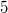$5$ Graphs from Elliptic Semiplanes

#### Abstract

For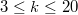$3 \le k \le 20$ with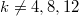$k \ne 4,8,12$, all the smallestcurrently known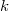$k$--regular  graphs of girth$5$ have the sameorders as the girth$5$ graphs obtained by the followingconstruction: take a (not necessarily Desarguesian) ellipticsemiplane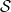$\cal S$ of order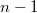$n-1$ where$n = k - r$ for some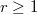$r\ge 1$; the Levi graph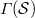$\varGamma({\cal S})$ of$\cal S$ is an$n$--regular graph of girth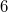$6$;  parallel classes of$\cal S$induce co--cliques in$\varGamma({\cal S})$, some of which areeventually deleted; the remaining co--cliques are amalgamated withsuitable$r$--regular graphs of girth at least$5$. For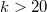$k > 20$,this construction yields some new instances underbidding thesmallest orders known so far.

DOI Code: 10.1285/i15900932v29n1supplp91

Keywords:
(k,5)-cages; girth 5 graphs; elliptic semiplanes; Hughes planes

Full Text: PDF